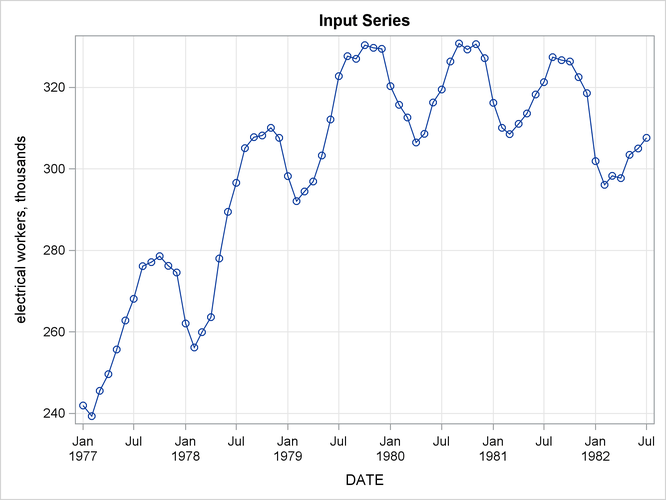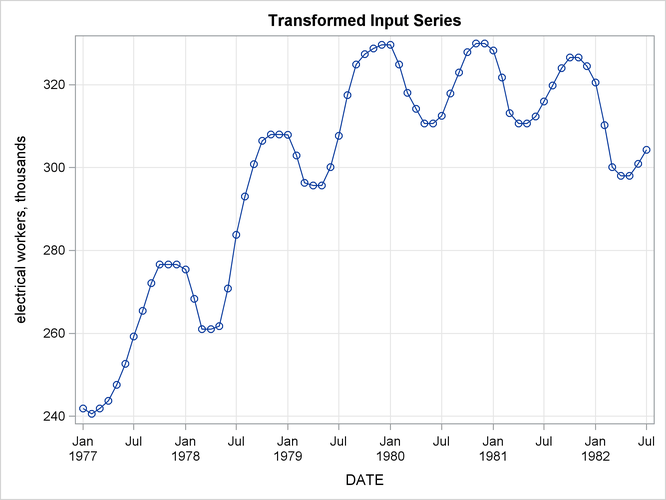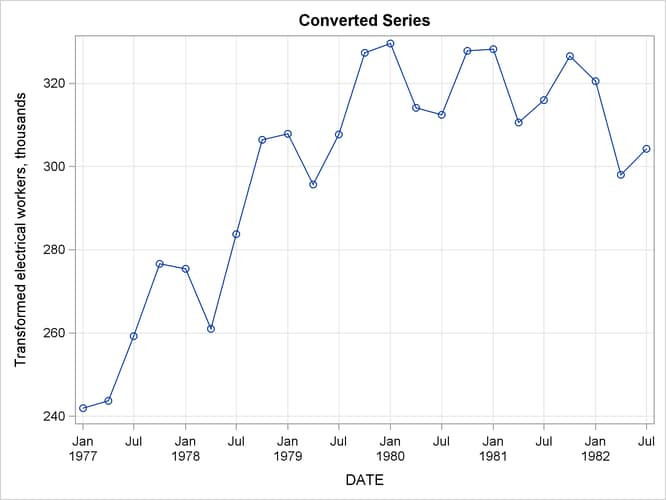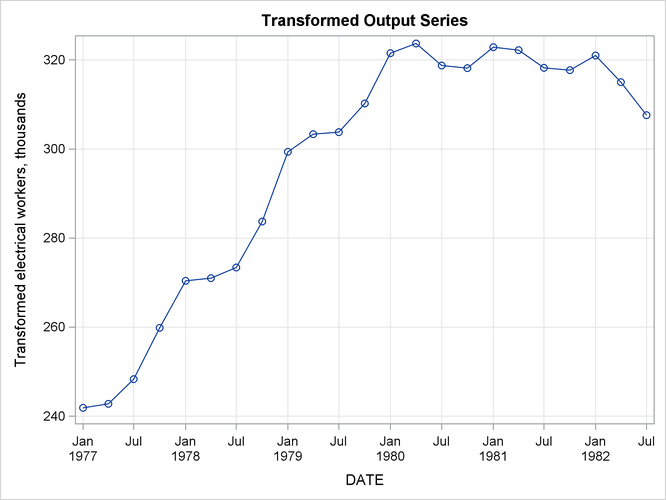# The EXPAND Procedure

### Example 15.2 Illustration of ODS Graphics

This example illustrates the use of ODS graphics with PROC EXPAND.

The graphical displays are requested by specifying the PLOTS= option in the PROC EXPAND statement. For information about the graphics available in the EXPAND procedure, see the section ODS Graphics.

The following statements utilize the `SASHELP.WORKERS` data set to convert the time series of electrical workers from monthly to quarterly frequency and display ODS graphics plots. The PLOTS=ALL option is specified to request the plots of the input series, the transformed input series, the converted series, and the transformed output series. Output 15.2.1 through Output 15.2.4 show these plots.

```proc expand data=sashelp.workers out=out
from=month to=qtr
plots=all;
id date;
convert electric=eout / method=spline
transformin=(movmed 4)
transformout=(movave 3);
run;
```

Output 15.2.1: Input Series PlotOutput 15.2.2: Transformed Input Series Plot—Four-Period Moving MedianOutput 15.2.3: Converted Plot of Transformed Input SeriesOutput 15.2.4: Transformed Output Series Plot—Three-Period Moving Average